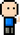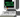# Josh Meets ComputerApril 22, 2017

# Image Processing for Lane Detection

The purpose of this journal is to explore image processing techniques for roadway images.

``````import matplotlib.pyplot as plt
import matplotlib.image as mpimg
import numpy as np
import cv2

%matplotlib inline
plt.rcParams['figure.figsize'] = (10.0, 8.0) # set default size of plots
plt.rcParams['image.interpolation'] = 'nearest'
plt.rcParams['image.cmap'] = 'gray'

# Grab size of image
y_size, x_size = image.shape[0:2]

# Create Copies
image_color_filter = np.copy(image)

# Original Image
plt.title('Original Image')
plt.imshow(image)``````
``<matplotlib.image.AxesImage at 0x231d45a9518>``

### Threshold Image by Color

Taking a full color image, mask all pixels (drop color values to zero) where the pixel intensities are not sufficient for the color white.

``````def color_mask(img, red=150, green=150, blue=0):
'''
Thresholds an image based on RGB color. Drops all pixels that does not meet this color threshold
input:
{image}: an image variable assigned by matplotlib.image.imread
output:
{region_selection}: an image with masking applied

'''
filtered_image = np.copy(img)
# Color Threshold
rgb_threshold = [red, green, blue]

# Reduce values below threshold
meets_threshold = (img[:, :, 0] < rgb_threshold) \
| (img[:, :, 1] < rgb_threshold) \
| (img[:, :, 2] < rgb_threshold)

filtered_image[meets_threshold] = [0, 0, 0]

return filtered_image
plt.title('Dropping colors')
``<matplotlib.image.AxesImage at 0x231db8e3278>``

### Segment Image by Region

Looking at the image above, turns out there are areas in the picture that contained colors matching the color filter, so they did not get masked by color thresholding. Here, the picture will be masked by a triangular region where the road should be.

``````def region_mask(image):
'''
Masks all portions of the image that does not include a road.

input:
{image}: an image variable assigned by matplotlib.image.imread
output:
{region_selection}: the same image with masking applieds

'''

# Pull out the x and y sizes and make a copy of the image
y_size = image.shape
x_size = image.shape
region_selection = np.copy(image)

point_A = [-350, y_size]
point_B = [x_size * 2/3, y_size * 3/10]
point_C = [x_size + 200, y_size]

# Create lines of triangular mask
line_AB = np.polyfit((point_A, point_B), (point_A, point_B), 1)
line_BC = np.polyfit((point_C, point_B), (point_C, point_B), 1)
line_AC = np.polyfit((point_A, point_C), (point_A, point_C), 1)

XX, YY = np.meshgrid(np.arange(0, x_size), np.arange(0, y_size))

# Thresholding the grid values within the region
threshold = (YY > (XX * line_AB + line_AB)) & \
(YY > (XX * line_BC + line_BC)) & \
(YY < (XX * line_AC + line_AC))
threshold = threshold != 1

# Color pixels red within this region
region_selection[threshold] = [0, 0, 0]

return region_selection
``<matplotlib.image.AxesImage at 0x231da7ccc18>``
``````# Process the image for both color and region
processed_image = np.copy(image)
plt.imshow(processed_image)``````
``<matplotlib.image.AxesImage at 0x231dc7e6550>``

### Canny Edge Detection

``````# Canny Filter parameters
low_threshold, high_threshold, kernel = 100, 400, 3

grayscale = cv2.cvtColor(np.copy(processed_image), cv2.COLOR_RGB2GRAY)
blur_gray = cv2.GaussianBlur(grayscale, (kernel, kernel), 0)
edge_only = cv2.Canny(blur_gray, low_threshold, high_threshold)

plt.title('Canny Edge detection Image')
plt.imshow(edge_only)``````
``<matplotlib.image.AxesImage at 0x231e6d182e8>``

### Hough Transform

``````# Define the Hough transform parameters
# Make a blank the same size as our image to draw on
rho = 1
theta = np.pi/180
threshold = 1
min_line_length = 10
max_line_gap = 1
line_image = np.copy(edge_only)*0 #creating a blank to draw lines on

# Run Hough on edge detected image
lines = cv2.HoughLinesP(edge_only, rho, theta, threshold, np.array([]),
min_line_length, max_line_gap)

# Iterate over the output "lines" and draw lines on the blank
for line in lines:
for x1,y1,x2,y2 in line:
cv2.line(line_image,(x1,y1),(x2,y2),(255,0,0),10)

# Create a "color" binary image to combine with line image
color_edges = np.dstack((edge_only, edge_only, edge_only))

# Draw the lines on the edge image
combo = cv2.addWeighted(color_edges, 0.8, line_image, 1, 0)
plt.imshow(combo)``````